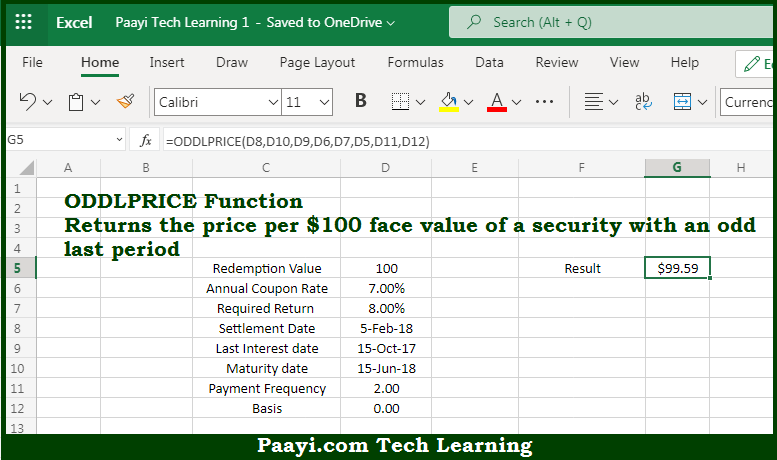# Learn How to Use Microsoft Excel ODDLPRICE Function

Written by | 0 Comments | 463 Views

In this article, you will learn how to use the Microsoft Excel ODDLPRICE function and its prime function in Microsoft Excel. You will also get to know the Microsoft Excel ODDLPRICE function return value and syntax with the help of some examples.

Microsoft Excel ODDLPRICE Function

The main purpose of the Microsoft Excel ODDLPRICE function is to get the price per \$100 face value where the last period will be odd. That implies, with the help of the ODDLPRICE function you can able to return the price per \$100 face value of a security with an odd or irregular last period. So, with the help of the ODDLPRICE function, you can able to get the price per \$100 face value where the last period will be odd.

Return Value of ODDLPRICE Function

The return value will be the bond price.

Syntax of ODDLPRICE Function

=ODDLPRICE(sd, md, id, rate, yield, redem, freq, [basis])

Where the arguments:

• sd - This is the settlement date of the security.
• md - This is the maturity date of the security.
• id - This is the issue date of the security.
• rate - This is the annual coupon rate of security.
• yield - This is the annual required rate of the return.
• redem - This is the redemption value per \$100 face value.
• freq - This is the coupon payments per year, where, annual = 1, semiannual = 2; quarterly = 4.
• basis - This is the day count basis, that's defaults to 0 (optional).

How to Use Microsoft Excel ODDLPRICE Function?So we know that Microsoft Excel ODDLPRICE function you can able to get the price per \$100 face value where the last period will be odd. That implies, with the help of the ODDLPRICE function you can able to return the price per \$100 face value of a security with an odd or irregular last period. So, with the help of the ODDLPRICE function, you can able to get the price per \$100 face value where the last period will be odd.